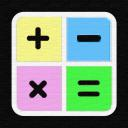## Math Basic

119 Members 24 Online Last Bump: 5 hours ago

#### Introduction

1 + 1 = ? if you know the answer of this equation, then you are welcome a place for learning mathematics, geometry, algorithms and whatever related to it

#### Description

1 + 1 = ? if you know the answer of this equation, then you are welcome a place for learning mathematics, geometry, algorithms and whatever related to it we also try to have video tutorials per week daily voice chat and sharing knowledge and a lot more

<-Education->